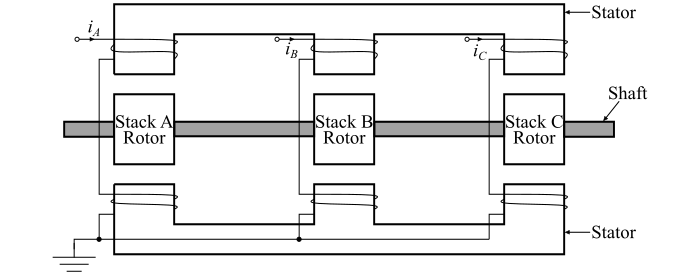# What is a Multi-Stack Variable Reluctance Stepper Motor?

Electronics & ElectricalElectronDigital Electronics

A multi-stack variable reluctance stepper motor having m-stacks can be considered to be made of m identical single-stack variable reluctance stepper motors with their rotors mounted on a single shaft.

In the multi-stack variable reluctance stepper motor, the stators and the rotors have the same number of poles or teeth and hence the same pole pitch. Therefore, for an m-stack stepper motor, the stator poles in all m-stacks are aligned, but the rotor poles are displaced by $(\frac{1}{m})$ of the pole pitch angle from one another.

All the stator pole windings in a given stack being energised simultaneously and thus, the stator winding of each stack forms one phase. Hence, the stepper motor has the same number of phases as the number of stacks.The cross-sectional view of a three-stack variable reluctance stepper motor is shown in the figure. Let, in each stack of the given stepper motor, stators and rotors have 12 poles. For a 12-pole rotor, the pole pitch is being 30 and hence, the rotor poles are displaced from each other by $(\frac{1}{3})$ of the pole pitch i.e. by 10°. The stator teeth in each stack are aligned.

When the phase winding A is energised, the rotor teeth of stack A are aligned with the stator teeth. When phase winding B is excited and the phase winding A is de-energised, the rotor teeth of the stack B are aligned with the stator teeth. This new alignment is made by the rotor movement of 10° in the anti-clockwise direction. Hence, the rotor moves one step due to change of the excitation from stack A to stack B.

Next, the phase C is excited and the phase B is de-energised, then the rotor moves by another step of $(\frac{1}{3})$ of pole pitch in the anti-clockwise direction. Further change of excitation from stack C to stack A will once more align the stator and rotor teeth in the stack A.

For a multi-stack variable reluctance stepper motor, if Nr is the number of rotor teeth and m is the number of stacks or phases, then

$$\mathrm{Tooth\:or\:pole\:pitch =\frac{360°}{𝑁_{𝑟}}}$$

And the step angle is

$$\mathrm{Step\:angle =\frac{360°}{𝑚\:𝑁_{𝑟}}}$$

In the above case,

$$\mathrm{Tooth\:or\:pole\:pitch =\frac{360°}{𝑁_{𝑟}}=\frac{360°}{12}= 30°}$$

$$\mathrm{Step\:angle =\frac{360°}{m\:𝑁_{𝑟}}=\frac{360°}{3×12}= 10°}$$

Multi-stack variable reluctance stepper motors are mainly used to obtain smaller step sizes (in the range of 2° to 15°). The multi-stack variable reluctance stepper motors have high torque to inertia ratio. The reduced inertia enables the stepper motor to accelerate the load faster.